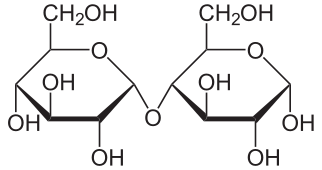# What monosaccharides are in maltose?

Jun 27, 2015

There is only one monosaccharide in sucrose, and that is glucose.

#### Explanation:

The structure of glucose, ${\text{C"_6"H"_12"O}}_{6}$, isThe $\text{OH}$ on $\text{C-1}$ of glucose can combine with the $\text{OH}$ on $\text{C-4}$ of another glucose molecule to form maltose.We could write the equation for the formation of maltose as

 underbrace(2"C"_6"H"_12"O"_6)_color(red)("glucose") → underbrace("C"_12"H"_22"O"_11)_color(red)("maltose") + "H"_2"O"